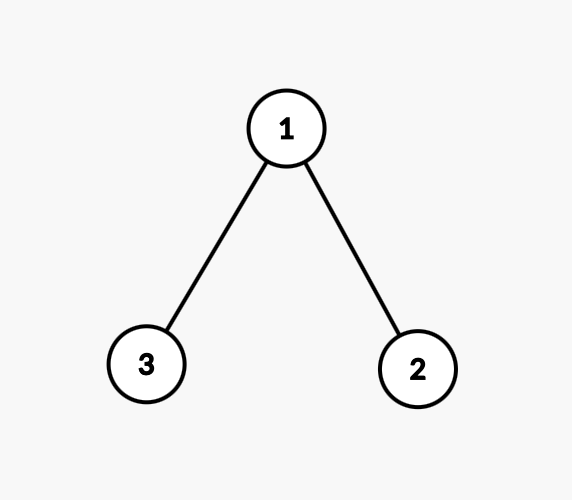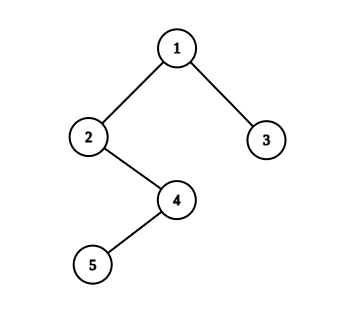# XOR Property

Posted: 13 Dec, 2020
Difficulty: Easy

## PROBLEM STATEMENT

#### XOR Property of Binary Tree: Every node should be XOR of it's left and right child.

##### For Example :
``````Consider the Binary Tree below :
````````````Here only node 1 has both its children. Also 1 = 3^2. So the answer for the above test case is true. We consider the xor only when both the children are present.
``````
##### Input Format :
``````The first line contains a single integer ‘T’ denoting the number of test cases. Then each test case follow.

The first line of each test case contains the elements of the binary tree in the level order form separated by a single space.

If any node does not have a left or right child, take -1 in its place. Refer to the example below.
````````````1
2 3
-1 4 -1 -1
5 -1
-1 -1

Explanation :
Level 1 :
The root node of the tree is 1

Level 2 :
Left child of 1 = 2
Right child of 1 = 3

Level 3 :
Left child of 2 = null (-1)
Right child of 2 = 4
Left child of 3 = null (-1)
Right child of 3 = null (-1)

Level 4 :
Left child of 4 = 5
Right child of 4 = null (-1)

Level 5 :
Left child of 5 = null (-1)
Right child of 5 = null (-1)

The first not-null node (of the previous level) is treated as the parent of the first two nodes of the current level. The second not-null node (of the previous level) is treated as the parent node for the next two nodes of the current level and so on.

The input ends when all nodes at the last level are null (-1).

Note: The above format was just to provide clarity on how the input is formed for a given tree.

The sequence will be put together in a single line separated by a single space. Hence, for the above-depicted tree, the input will be given as:

1 2 3 -1 4 -1 -1 5 -1 -1 -1
``````
##### Output Format :
``````For each test case, return True or False denoting whether the given binary tree satisfies the above condition.
Output for each test case will be printed in a separate line.
``````
##### Note :
``````You are not required to print anything; it has already been taken care of. Just implement the function.
``````
##### Constraints :
``````1 <= T <= 10
1 <= number of nodes <= 5000
1 <= nodeVal <= 10^9

Time Limit: 1 se
``````Approach 1

We try to visit every node in the tree using any traversal like dfs. We check if all nodes in the subtree of the current node satisfy the xor property and return true and false accordingly. We return true only when both the children return true as well as the current node satisfies the property.

Algorithm:

1. Create a recursive function checkXorProperty that takes a node as input and whether all nodes of this tree satisfy the xor property.
2. If we reach a null node return true as no nodes are present here.
3. Call the function for left subtree and right subtree recursively.
4. If both the right and left children are present we check if their xor is equal to the current node or not. If not, we return false immediately.
5. Else we return the result as the logical ‘and’ of results of left and right children.